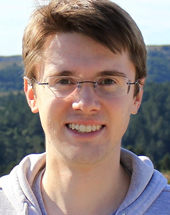Diophantine Problems and $$p$$-adic Period Mappings (after Lawrence and Venkatesh) (Fall 2021)

The goal of this seminar is to go over the recent proof of Faltings's theorem by Lawrence and Venkatesh [LV]. The method of this proof, which heavily involves $$p$$-adic Hodge theory rather than heights and abelian varieties, may also be applied to higher dimensional varieties. We will follow the program outlined here.

• Organizers: Caleb Ji and Ivan Zelich
• When: Friday 12:15 pm - 1:30 pm
• Where: Room 528
• References:
[LV] Brian Lawrence and Akshay Venkatesh Diophantine problems and $$p$$-adic period mappings https://arxiv.org/abs/1807.02721
• Notes from the seminar are here.

Schedule

Sept 24
Caleb Ji
Faltings's theorem: comparison of approaches
I will give an overview of both Faltings's original proof of the Mordell conjecture and the Chabauty-Kim approach. Then I will outline the strategy of the proof given by Lawrence and Venkatesh.
Oct 1
Caleb Ji
The Gauss-Manin connection and the period mapping
The complex period mapping is important for the Lawrence-Venkatesh proof of Faltings's theorem not only because it motivates the p-adic version, but also because it is directly used for computations. In this talk, I will explain this subject beginning from the correspondence between local systems and representations of the fundamental group. In the complex analytic scenario, these also correspond to holomorphic vector bundles with flat connections known as the Gauss-Manin connection. The upshot for us is that the complex cohomology of fibers is locally constant. However, the period map encodes the variation of the Hodge filtration on these cohomology groups. I will illustrate this theory with the classical example of the Picard-Fuchs equation.
Oct 8
Ivan Zelich
Algebraic de Rham cohomology and crystalline cohomology
We give a brisk introduction to algebraic De Rham cohomology and how the Gauss-Manin connection naturally arises from a spectral sequence used to compute it.
Oct 15
Ivan Zelich
PD structures
We begin by giving more details on the algebraic Gauss-Manin connection mentioned last time. Then we describe on PD structures remedy the lack of a Poincaré lemma in the p-adic setting, which will lead to the construction of crystalline cohomology.
Oct 22
Ivan Zelich
Crystalline cohomology
We use PD-thickenings to define the crystalline site, which allows us to define crystals and crystalline cohomology.
Oct 29
Caleb Ji
We begin by reviewing the theory of local fields and l-adic representations of $$\operatorname{Gal}(K)$$, where $$K$$ is a local field with residue field characteristic $$p$$. We then state and explain the basic theorems of p-adic Hodge theory through Fontaine's period rings, which describe properties of $$p$$-adic representations coming from geometry. We end by sketching the construction of some of the period rings.
Nov 5
Caleb Ji
The S-unit equation
We return to the original problem of bounding rational points on varieties. We use the theory developed in the previous lectures (Gauss-Manin connection, period mappings, p-adic Hodge theory), to prove a general prototype result that exhibits the strategy of this method. We also apply this method to the S-unit equation, proving that the number of pairs of $$S$$-integers in some number field $$u, v\in \mathcal{O}_S$$ satisfying $$u+v=1$$ is finite.
Nov 12
Caleb Ji
Proof of Faltings's theorem modulo facts about the Kodaira-Parshin family
We introduce the Kodaira-Parshin family, which is the underlying family used for the proof of Faltings's theorem. Assuming various results about it which will be covered in future talks, we prove Faltings's theorem.
Nov 19
Caleb Ji
Construction of the Kodaira-Parshin family
We introduce Hurwitz spaces and Prym varieties and use them to construct the Kodaira-Parshin family. Along the way, we discuss some alternative approaches to studying branched covers of $$\mathbb{P}^1$$, namely through dessins d'enfants.
Nov 25
Thanksgiving - no talk
Dec 3
Caleb Ji
Monodromy of the Kodaira-Parshin family
I will begin by introducing the basic theory of mapping class groups. Then I will use them to outline a proof that the Kodaira-Parshin family has full monodromy. As we have seen eariler, this is a key property of the Kodaira-Parshin family which leads to a proof of Faltings's theorem.
Dec 10
Caleb Ji
Higher dimensions and o-minimality
I will begin by giving an introduction to o-minimality and its application to transcendence questions. Then I will explain how these ideas can be applied in the context of variations of Hodge structures. A p-adic version of these results is used by Lawrence and Venkatesh to higher dimensional cases of the Shafarevich conjecture.